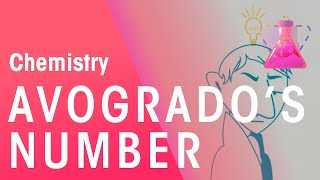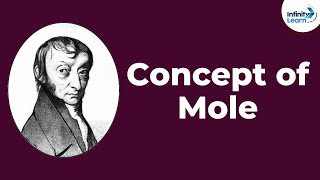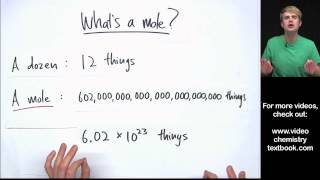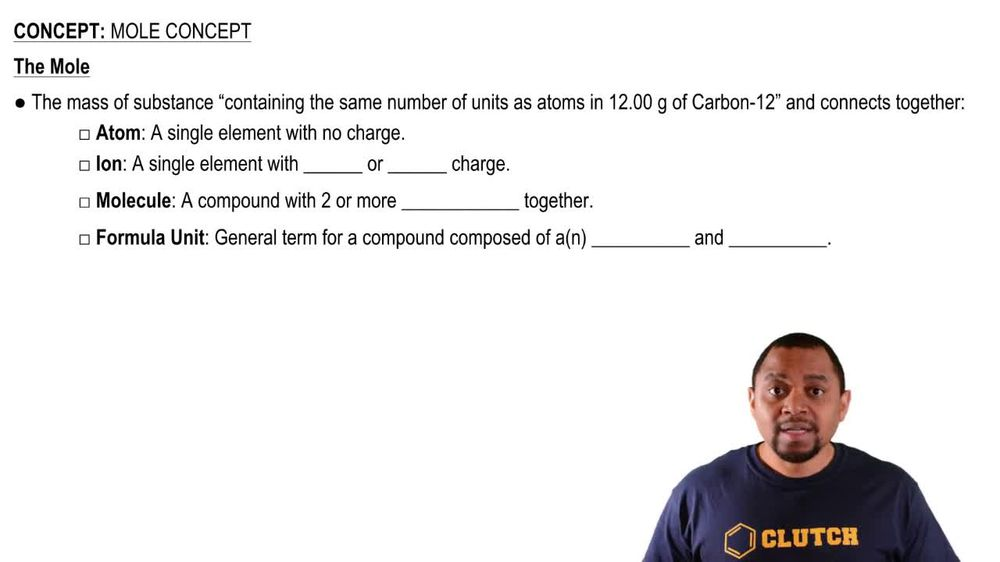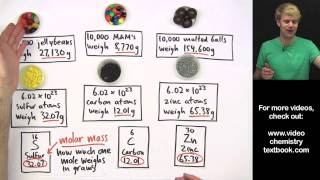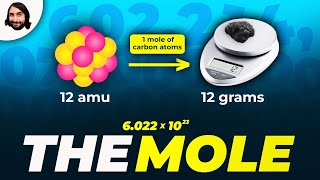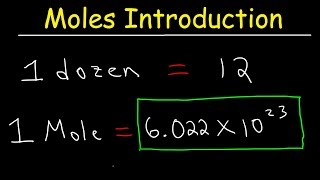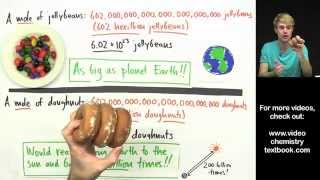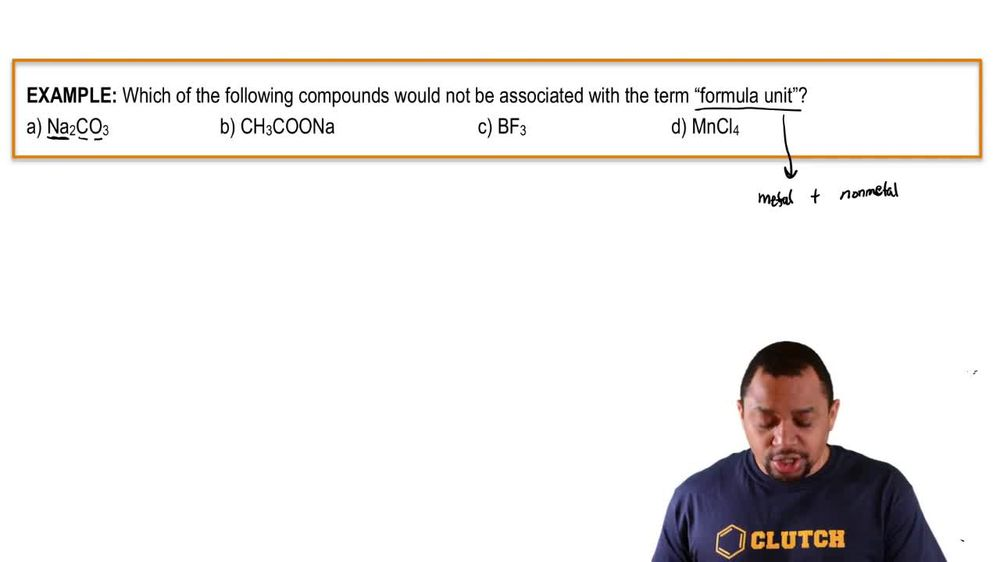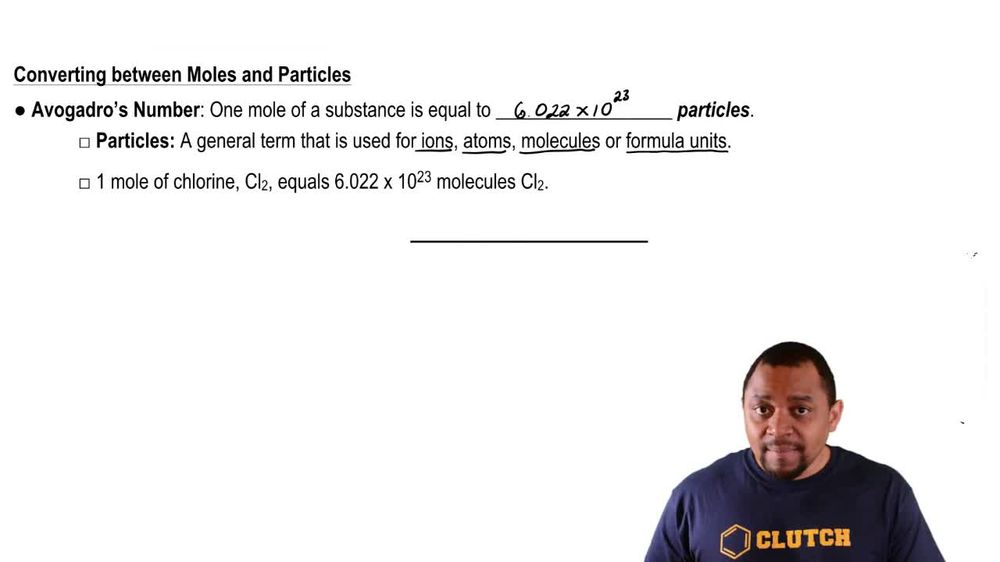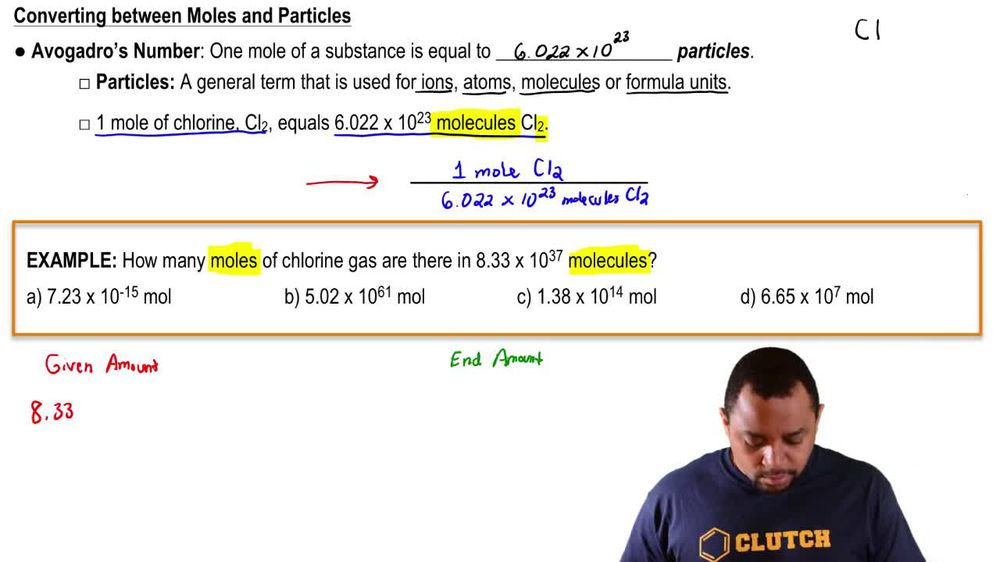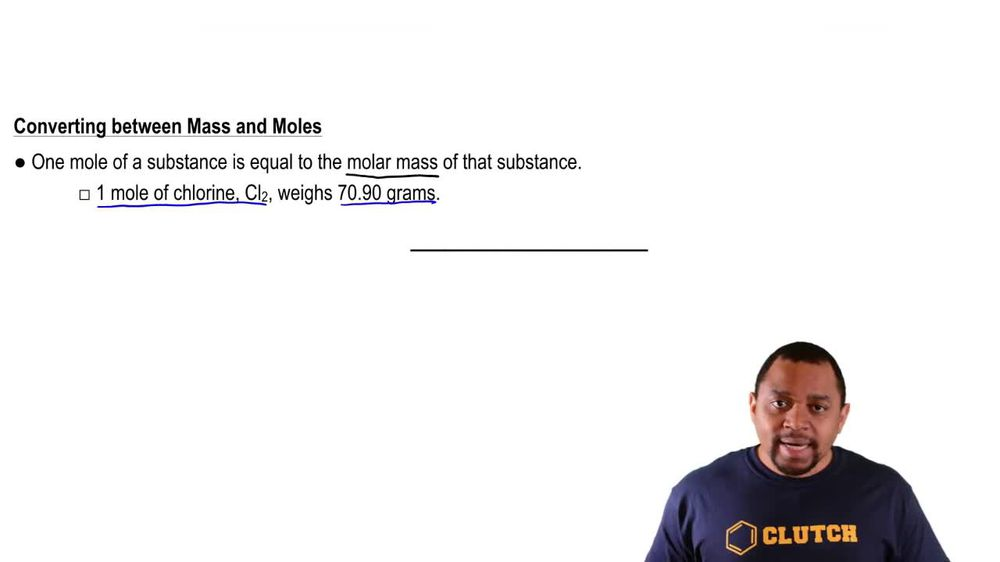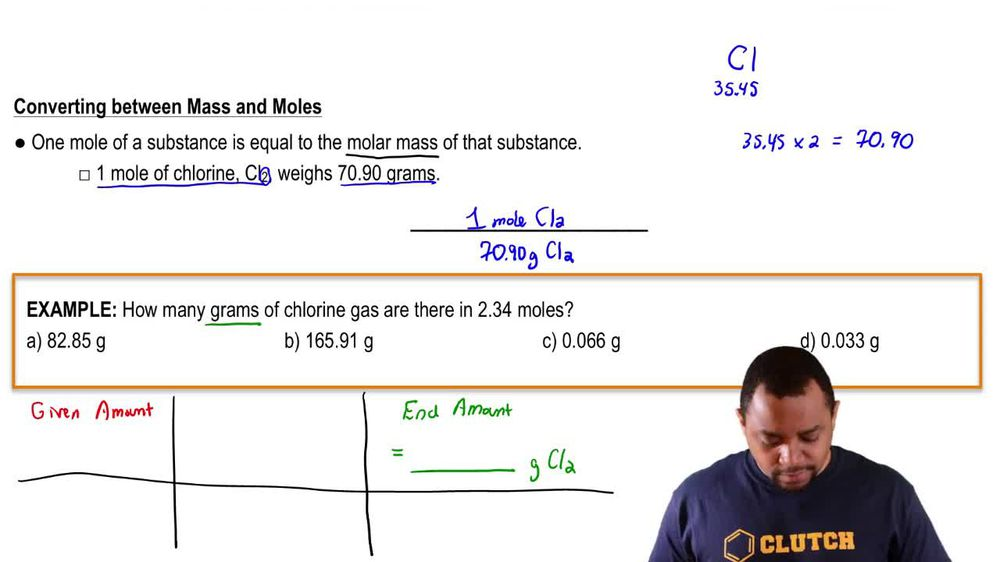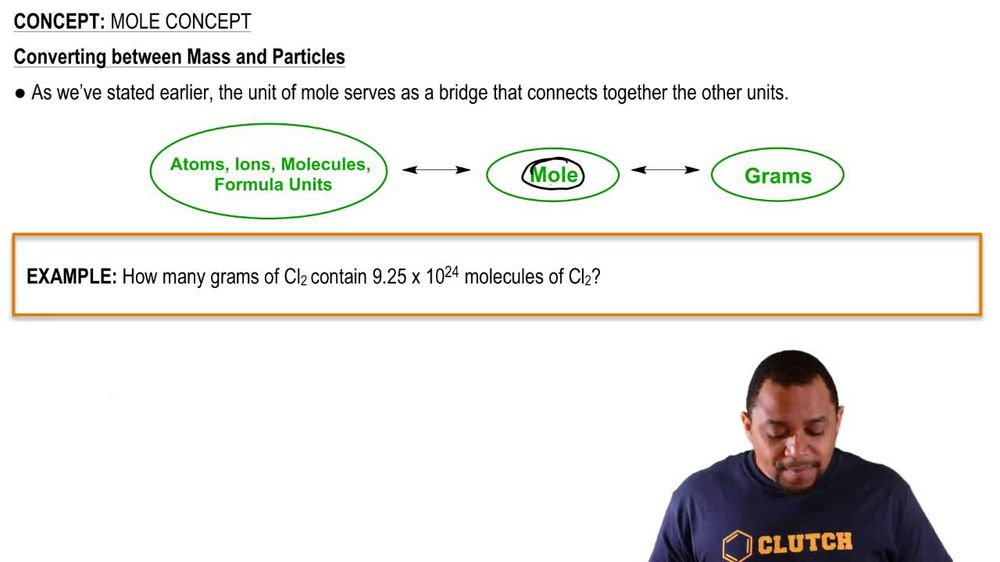Start typing, then use the up and down arrows to select an option from the list.
1. 2. Atoms & Elements2. Mole Concept
Problem

# A cube of gold that is 1.00 cm on a side has a mass of 19.3 g. A single gold atom has a mass of 197.0 u. (a) How many gold atoms are in the cube?

Relevant Solution1m
Play a video:
Hi everyone we here we have a question telling us that a titanium cube has sides that measure 2.50 centimeters and has a massive 28.1 g. Our goal is to calculate the number of titanium atoms in the cube if the single titanium atom has a mass of 47.8 atomic mass units. So we're starting off with our 28.1 g And we're multiplying by one mole and we know that one mole has a mass of 47.8 g from our prompt. And then we're gonna multiply that by avocados number. So 6.02, 2 times 10 to the 23rd atoms. And that is in one mole, so divided by one mole. So our grams are canceling out and our molds are canceling out, leaving us with 3.54 Times 10 to the 23rd atoms. And that is our final answer. Thank you for watching. Bye.